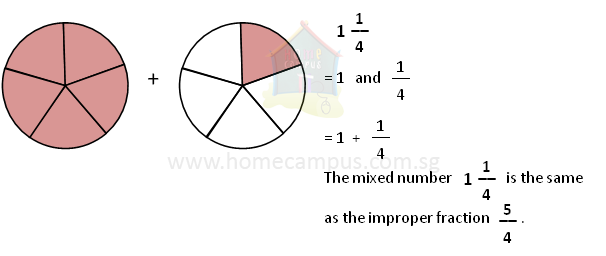## Types of Fractions?

Practice Unlimited Questions

#### 1. What are the different types of fractions?

There are 3 different types of fractions:
1. Proper fractions
2. Improper fractions
3. Mixed numbers or mixed fractions

#### 2. What are Proper Fractions?

In a proper fraction, the numerator (top number) is smaller than the denominator (bottom number).

Examples:
 3 4
,
 4 5
,
 12 17
,  etc.#### 3. What are Improper Fractions?

In an improper fraction, the numerator (top number) is bigger than or equal to the denominator (bottom number).

Examples:
 4 3
,
 6 5
,
 10 7
,
 4 4
,  etc.#### 4. What are Mixed Numbers or Mixed Fractions?

A mixed number is made up of two parts - a whole number and a proper fraction.

Examples:
 5 2 3
,
 1 1 4
,
 3 5 12
,
 2 1 2
,  etc.#### Notes:

• Improper fractions can be converted to mixed numbers and mixed numbers can be converted back to improper fractions. Improper fractions and mixed numbers are 2 different ways to represent the same number.

• Improper fractions are useful when writing mathematical formulae. Compared to a mixed number, it's easier to write, use and operate upon a number written as an improper fraction and not be confused.

• Mixed numbers have their utility when talking about quantities in day-to-day life. For example, you say,
"I need  2 1 2
cups of sugar for the cake."